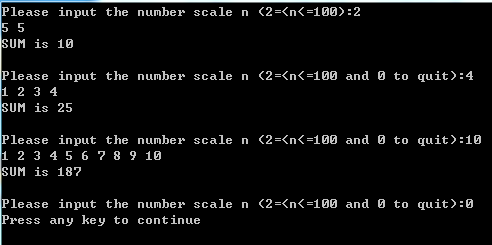2015-11-08 04:16

# 小弟不才，求完整代码

• 写回答
• 好问题 提建议
• 关注问题
• 收藏
• 邀请回答

#### 4条回答默认 最新

•Meditator_hkx 2015-11-08 07:20
已采纳

// 20151108csdnacm.cpp : Defines the entry point for the console application.
//

#include "stdafx.h"
#include "stdio.h"
#define MAX_N 100
#define MAX_COMB 100*99/2

int repeat_del(int a[],int n);
int final_sum(int a[],int n);

int main(int argc, char* argv[])
{
int n = 1,pair_num,final_num,sum;
int i;
printf("Please input the number scale n (2=<n<=100):");
scanf("%d",&n);
while ( n != 0) {
int a[MAX_N];
for(i = 0;i < n;i++) { //Input the numbers (and we can add error handling if it's needed）.
scanf("%d",&a[i]); //cannot written as a[i] here.
}
printf("SUM is %d\n",sum);

``````    printf("\nPlease input the number scale n (2=<n<=100 and 0 to quit):");
scanf("%d",&n);
}
return 0;
``````

}

int pair_add(int a[],int n,int b[]) {
int i,j,k = 0;
for (i = 0;i < n-1;i++) {
for (j = i+1;j < n;j++) {
b[k] = a[i] + a[j];
k++;
}
}
return k;
}

int repeat_del(int a[],int n) {
int i,j,k;
for (i = 0;i < n-1;i++) {
for (j = i+1;j < n;j++) {
if (a[i] == a[j]) {
for (k = j;k < n-1;k++)
a[k] = a[k+1];
n--;
j--;
}
}
}
return n;
}

int final_sum(int a[],int n) {
int sum = 0,i;
for(i = 0;i < n;i++)
sum += a[i];
return sum;
}

已采纳该答案
评论
解决 无用
打赏 举报
•weixin_32564957 2015-11-08 04:16

Description
Small W is playing a summary game. Firstly, He takes N numbers. Secondly he takes out every pair of them and add this two numbers, thus he can get N*(N - 1)/2 new numbers. Thirdly he deletes the repeated number of the new numbers. Finally he gets the sum of the left numbers. Now small W want you to tell him what is the final sum.

Input
Multi test cases, every case occupies two lines, the first line contain n, then second line contain n numbers a 1, a 2, ……a n separated by exact one space. Process to the end of file.
[Technical Specification]
2 <= n <= 100
-1000000000 <= a i <= 1000000000

Output
For each case, output the final sum.

Sample Input
4
1 2 3 4
2
5 5

Sample Output
25
10
Hint
Firstly small W takes any pair of 1 2 3 4 and add them, he will get 3 4 5 5 6 7. Then he deletes the repeated numbers, he will get 3 4 5 6 7, Finally he gets the sum=3+4+5+6+7=25
附上文字版

评论
解决 无用
打赏 举报
•Meditator_hkx 2015-11-08 06:55

不是难题，但是我C语言有点生疏了，很多地方处理的不是很快，附上经过验证的代码给题主：

`````` #include "stdafx.h"
#include "stdio.h"
#define MAX_N 100
#define MAX_COMB 100*99/2

int repeat_del(int a[],int n);
int final_sum(int a[],int n);

int main(int argc, char* argv[])
{
int n = 1,pair_num,final_num,sum;
int i;
printf("Please input the number scale n (2=<n<=100):");
scanf("%d",&n);
while ( n != 0) {
int a[MAX_N];
for(i = 0;i < n;i++) {      //Input the numbers (and we can add error handling if it's needed.
scanf("%d",&a[i]);      //cannot written as a[i] here.
}
printf("SUM is %d\n",sum);

printf("\nPlease input the number scale n (2=<n<=100 and 0 to quit):");
scanf("%d",&n);
}
return 0;
}

int pair_add(int a[],int n,int b[]) {
int i,j,k = 0;
for (i = 0;i < n-1;i++) {
for (j = i+1;j < n;j++) {
b[k] = a[i] + a[j];
k++;
}
}
return k;
}

int repeat_del(int a[],int n) {
int i,j,k;
for (i = 0;i < n-1;i++) {
for (j = i+1;j < n;j++) {
if (a[i] == a[j]) {
for (k = j;k < n-1;k++)
a[k] = a[k+1];
n--;
j--;
}
}
}
return n;
}

int final_sum(int a[],int n) {
int sum = 0,i;
for(i = 0;i < n;i++)
sum += a[i];
return sum;
}
``````

你可以把printf语句去掉以符合题意要求，我是为了测试方便才做的while循环，你也可以删去。

评论
解决 无用
打赏 举报
•Meditator_hkx 2015-11-08 06:57这个是结果显示图。
题主如果觉得哪些地方费解或者冗余可以反馈给我，也是对我的一个提醒。
望采纳~

评论
解决 无用
打赏 举报